## InfinityCodeX

Check out our blogs where we cover topics such as Python, Data Science, Machine Learning, Deep Learning. A Best place to start your AI career for beginner, intermediate peoples.

## Classify Infected Malaria Cell Using CNN Python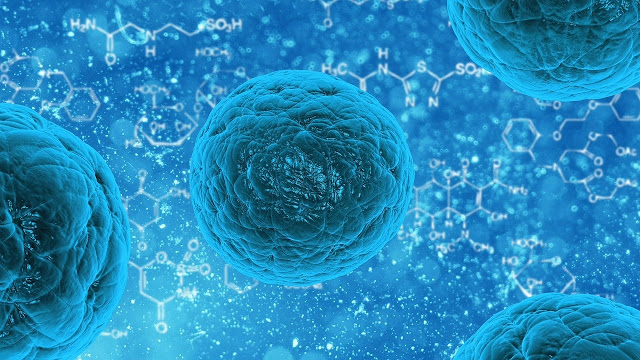CELL

In this article, we will look at end-to-end real-life Convolutional Neural Network (CNN) project where we will detect the cell is infected by malaria or not. We will be using Python, Keras, and TensorFlow for this project.

The data set which is used in this project is taken from the National Library Of Medicine.National Library Of Medicine

This dataset contains multiple image’s of infected cells called as (‘parasitized’) :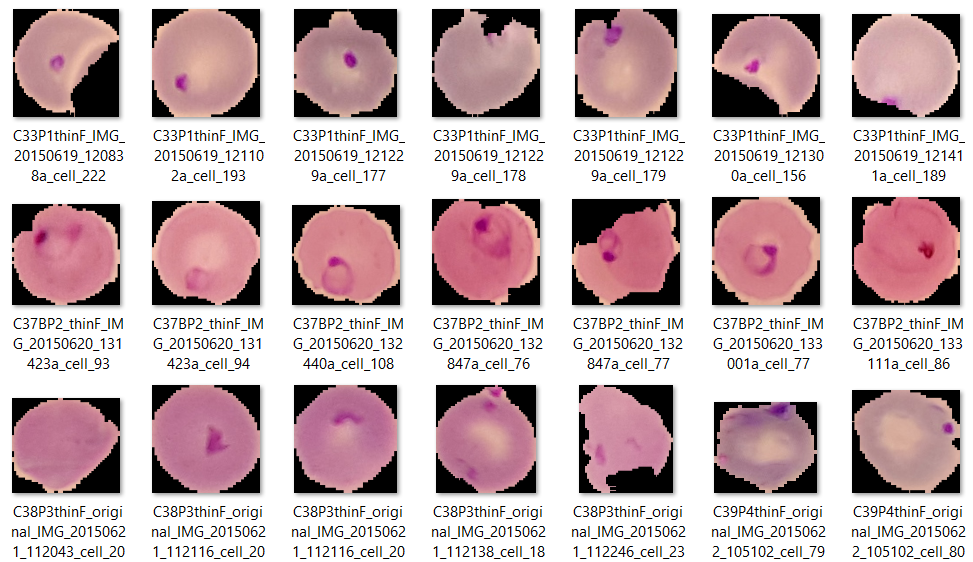INFECTED CELL

And multiple images of non-infected cells called as (‘uninfected’) :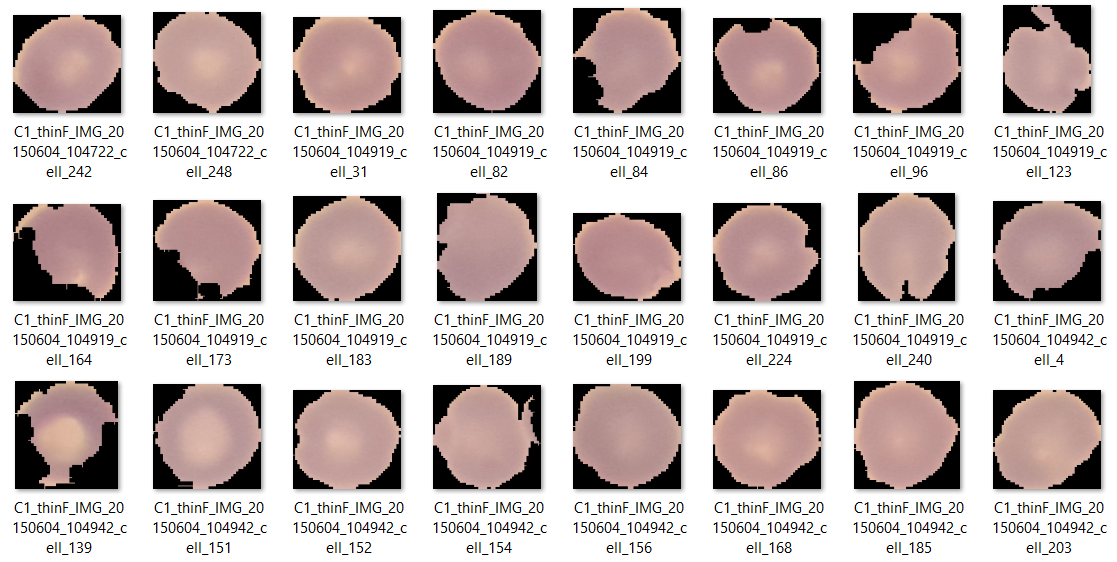Non-Infected Cells

So what we end up doing is attempting to build a model that just based on the image of a cell can predict whether or not it’s infected or not infected with malaria.

In real life, this may save doctors a lot of time by just running images into our model instead of having to manually look at these image’s themselves and determinant.

So now you can download this data from the official site or just click this link :

Now as you all have downloaded and extracted the data. Now it's time to execute some code.

Open your Jupyter-Notebook and let's begin.

The very first thing we will do is to take a look at the content which is present inside the folder.

import os
data = "D:\corona\CNN_data\cell_images"
os.listdir(data)

Output:

['test', 'train']

Now we will be importing some of the important Python libraries which will help us to visualize and manage the images.

import pandas as pd
import numpy as np
import seaborn as sns
import matplotlib.pyplot as plt
%matplotlib inline

Now to read the images which are present in our file we will import imread function which is present in the matplotlib library.

As we saw earlier that we have a test set and training set inside of this. Let's go and set 2 variables the first variable will be called test_path and what we are going to do here is that we are going to concatenate our data path with test and for the second one will be called as train_path and we will do the same thing with that too.

test_path = data + "\\test\\"
train_path = data + "\\train\\"

Now to read the images which are present in our file we will import imread function which is present in the matplotlib library.

The purpose of doing this is to see what images are present inside that path. To check the path again just type test_path and it will show you the path

test_path

Output:

'D:\\corona\\CNN_data\\cell_images\\test\\'

So now let's go ahead and list the files what kind of files are inside.

os.listdir(test_path)

Output:

['parasitized', 'uninfected']

If you noticed that this data set contains 2 folders which are parasitized and uninfected which we saw above. These folders contains about 27000 images and the state asset is taken from a government repository on malaria data sets.

So as I mentioned earlier we are going to end up doing is attempting to build a model that just based off the image of a cell can predict whether or not that cell is infected or not infected with malaria.

So now we are going to look at the single image.

os.listdir(train_path+'parasitized')

Output:

'C100P61ThinF_IMG_20150918_144104_cell_162.png'

Now let's visualize the single-cell image.

inf_cell = train_path+'parasitized\\'+'C100P61ThinF_IMG_20150918_144104_cell_162.png'

Output:

As we saw the infected cell above, now let's have a look at the non-infected cell.

os.listdir(train_path+'uninfected')

Output:

'C100P61ThinF_IMG_20150918_144104_cell_128.png'

uninf_cell = train_path+'uninfected\\'+'C100P61ThinF_IMG_20150918_144104_cell_128.png'

Output:

Now let's go ahead and finally figure out the average shape of, one of these images. The important thing about this dataset is that the images which are present are real images and it's unlikely that they all are going to be the exact same shape. If we compare this dataset with other datasets such as MNIST or CIFAR dataset you have noticed that every image in this dataset has the same dimensions. But in the real dataset, it's another way around they going to have different dimensions.

There are lots of different ways we can do this but one way is to set up 2 list dim1 and dim2 than we will be applying a for loop where we will be iterating through every file and by chance the test path uninfected. Then we are going to check the shape of each of them and then append their first dimension (dim1) and second dimension (dim2). Now let's code this theory.

dim1 = []
dim2 = []

for img_filename in os.listdir(test_path+'uninfected'):
d1,d2,color = img.shape
dim1.append(d1)
dim2.append(d2)

Now let's visualize the dimensions of dim1 and dim2.

sns.jointplot(dim1,dim2)

Output:

So why is this important?

Well, the Convolutional Neural Network isn't going to be able to train on images of various sizes. So what we need to do is to make sure that, we are going to resize all the images to be the same size. So we have to choose what is the actual dimensions should we resize everything to. The answer is pretty simple we should choose is essentially the average of both dimensions and this shows you the actual distribution of the images and they all kind of center around basically 130 by 130 and you can confirm this by checking out the mean values in your dimensions.

np.mean(dim1)

Output:

130.925

np.mean(dim2)

Output:

130.750

So now we can say that our final image shape which we will be feeding in our Convolutional Neural Network is 130 by 130 by 3

img_shape=(130,130,3)

Go ahead and run that. And then later on we are actually preparing the data for the model we will be resize everything to these dimensions. So if it's a smaller photo we'll basically add padding so that it reaches these dimensions and if it's a larger photo we can either crop, shrink, or compress that image.

Now we are going to focus on this special object from TensorFlow Keras which is called the image data generator. What we are going to do of this class is that we are going to feed it in the directory over our actual image files are and you'll be able to perform a bunch of manipulations on our images and then feed those new images to your model.

Before diving into the concept keep in mind that there is really too much data for us to read in all this data at once. This file is much larger than the files such as MNIST and CIFAR. If you know the dimension of MNIST is 28 by 28 and CIFAR has the dimension of 32 by 32, and even that the smaller expansion from 28 by 28 to color images of 32 by 32 was a huge expansion in the amount of data if we look at the math i.e 28*28 = 784 data points and when we see CIFAR that was 32*32*3 = 3072 and our files are going to be even larger that is 130*130*3 = 50700. Now we are dealing with 50700 data points because we are not going to able to just feed in everything at once. Instead, we have to select batches of our images. The other idea that we want to be able to overcome is the fact that it should be robust enough to deal with images that are pretty different from images that it's seen before and one way we can do that is by manipulating and performing transformations on our images thing like rotation, resizing and scaling.

Okay enough with the talk now let's go ahead and explore this idea of manipulating images as well as flowing from a directory these new batches of files.

from tensorflow.keras.preprocessing.image import ImageDataGenerator

Go ahead and run that command. If you had ever worked with MNIST dataset we had 60000 images and that's a lot of images for a very simple file type essentially a very simple image that had a dimension of 28 by 28. Right now we have half of that size over our entire dataset. Our entire dataset is less than 30000 images. So want to do is to expand the number of images without having gathered more data. We can't just keep grabbing blood cells from people. So we can do things like take our current images and randomly rotate them, reshape them, rescale them, etc.

from tensorflow.keras.preprocessing.image import ImageDataGenerator

img_gen = ImageDataGenerator(rotation_range=20,
width_shift_range=0.1,
height_shift_range=0.1,
shear_range=0.1,            #cutting part of image
zoom_range=0.1,
horizontal_flip=True,
fill_mode='nearest')       #fill data in image voids

Output:

plt.imshow((para_img))

Output:

Now let's have a look at our manipulated image.

plt.imshow(img_gen.random_transform(para_img))

Output:

So here is our randomized version of the image. Notice that we got to stretch, kind of like columns sticking out of the cell and that's because through this random transformation it looks like it got stretched out and fill in those values with the nearest pixel values and then notes it was also rotated makes a lot of sense to randomly rotate image here because their cell's that can be in any sort of rotational axis that they want. They can be floating around in their sample so depending again on the actual type of images you're looking at you're going to be playing around at these actual range values.

Okay so now with the fact that we can randomly transform these images we can essentially augment our dataset. We no longer restricted to just a single image from the cell we can randomly transform this many times over. So if we keep running this we'll see more and more random transformations. And this is the way artificially expanding our image dataset. Recall we have less than 30000 images but now we could do the random transformation on all those images and immediately double the size of our image dataset. Maybe we can do 5 random transformations and we went from something like 20000 images to 100000 images.

So this is the really powerful tool you have to keep in mind when you are dealing with kinda smaller datasets and when it comes to the Convolution Neural Networks it takes thousands and thousands of images to get something that performs really well.

plt.imshow(img_gen.random_transform(para_img))

Output:

Okay now, how do we actually set up our directories to flow batches from the directory. The way we do that by using the flow from the directory function.

img_gen.flow_from_directory(train_path)

Output:

Found 24958 images belonging to 2 classes.

<keras_preprocessing.image.directory_iterator.DirectoryIterator at 0x1f9aabb8808>

When you run that, you saw some text. Now, what that text means? It means that our machine had found around 24958 number of images belonging to 2 classes. There are 2 folders in the directory which indicates each class, that's how our machine is able to distinguish between classes. This would be the same for the test_path but at test number of images would be 2600.

IMP:  In order to use ".flow_from_directory", you must organize the image is sub-directories. This is an absolute requirement, otherwise, the method won't work. The directories should only contain images of one class, so one folder per class of images.

Okay now as we have learned about our dataset. Now it's time to create our model.

from tensorflow.keras.models import Sequential
from tensorflow.keras.layers import Dense, Conv2D, MaxPool2D, Dropout, Flatten

model = Sequential()

kernel_size=(3,3),
input_shape=img_shape,
activation='relu'))

kernel_size=(3,3),
input_shape=img_shape,
activation='relu'))

kernel_size=(3,3),
input_shape=img_shape,
activation='relu'))

model.compile(loss='binary_crossentropy',
metrics=['accuracy'])

model.summary()

Output:

IMPORTANT POINTS:

* MaxPool2D and MaxPooling2D are aliases that mean there are multiple names for the same function.

* Because this is going to be a larger network we added Dropout to it. Which will help us to prevent overfitting.

* input_shape should be equal to the img_shape.

* Keep in mind if you choose an image shape that's too large especially if you are dealing with extremely large files your machine will run out of memory. That completely depends on your hardware.

* We added multiple Convolutional layers because the larger the image size the more complex of a task you are dealing with more Convolutional layer you should have.

* As we go deeper the network we increase the number of filters.

* Dropout value is 0.5 because we want to turn off half of the neurons to prevent overfitting.

Notice that we have 1605760 number of parameters by the time we get to the dense layer. So this model will take a long time to train.

To make sure we can pick the right amount of epochs to train for we can use callbacks early stopping.

from tensorflow.keras.callbacks import EarlyStopping

early_stop = EarlyStopping(monitor='val_loss',patience=2)

batch_size = 16

train_img_gen = img_gen.flow_from_directory(train_path,
target_size=img_shape[:2],
color_mode='rgb',
batch_size=batch_size,
class_mode='binary')

test_img_gen = img_gen.flow_from_directory(test_path,
target_size=img_shape[:2],
color_mode='rgb',
batch_size=batch_size,
class_mode='binary',shuffle=False)

Output:

Found 24958 images belonging to 2 classes.

Found 2600 images belonging to 2 classes.

train_img_gen.class_indices

Output:

{'parasitized': 0, 'uninfected': 1}

Now we are going to train our model. Remember that it's going to take a lot of time to train.

results = model.fit_generator(train_img_gen,epochs=20,
validation_data=test_img_gen,
callbacks=[early_stop])

Or else we have an alternative for this which is very easy to implement.

Pre-Trained File:

model.summary()

Output:

The minor drawback with this is, you won't get the model history. But if you want you can evaluate the model.

model.evaluate_generator(test_img_gen)

Output:

[1.9182854999828034, 0.8738462]

That's amazing we got an accuracy of 87% which is pretty good considering that base accuracy is 50%.

Now let's see how accurate our model is using some prediction values.

pred = model.predict_generator(test_img_gen)

pred

Output:

array([[0.],
[0.],
[0.],
...,
[0.],
[1.],
[0.]], dtype=float32)

So if we take a look at the results here of pred we get the values between 0 and 1. So it actually doesn't return back the straight class calls, instead, it returns back the probabilities so you know that if the value of a point is 0.97 or 0.98 that means that our model is 97% or 98% sure that it belongs to class 1. So now what we will do is that if any value is greater than 0.5 i.e 50% it belongs to class 1 and if it's less than 0.5 it will belong to class 2.

predictions = pred > 0.5        # you can take any threshold value you want

predictions

Output:

array([[False],
[False],
[False],
...,
[False],
[ True],
[False]])

So True and False are treated as 0 and 1 in NumPy. So we can directly pass it through this to our confusion matrix and classification report.

If the value is 1 the cell is not infected and if the value is 0 it is infected. Look the class values {'parasitized': 0, 'uninfected': 1}.

from sklearn.metrics import classification_report,confusion_matrix

print(confusion_matrix(test_img_gen.classes, predictions))

Output:

[[1274   26]
[ 299   1001]]

from tensorflow.keras.preprocessing import image

Output:

my_img

Output: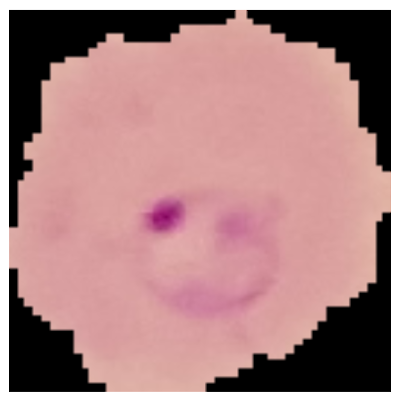Reshaped Malaria Infected Cell

So when we think about this as a real-world situation, what actually happens is that the doctor will give us the image and we going to load the image and pass it to the model.

my_img_array = image.img_to_array(my_img)
my_img_array.shape

Output:

(130, 130, 3)

So what we are going to do here is simply resize this along the 0 dimensions and we can do that in several ways, but one of the ways is we can use np.expand_size function.

my_img_array = np.expand_dims(my_img_array,axis=0)

my_img_array.shape   # We reshaped the image to 1 image of dimension 130,130,3

Output:

(1, 130, 130, 3)

model.predict(my_img_array)

Output:

array([[0.]], dtype=float32)

train_img_gen.class_indices

Output:

{'parasitized': 0, 'uninfected': 1}

This means that our model prediction is correct it is an infected cell.

So we hope that you enjoyed this project. If you did then please share it with your friends and spread this knowledge.

Instagram :

https://www.instagram.com/infinitycode_x/

Classify Malaria Using CNN Python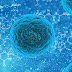Reviewed by InfinityCodeX on Saturday, June 27, 2020 Rating: 5